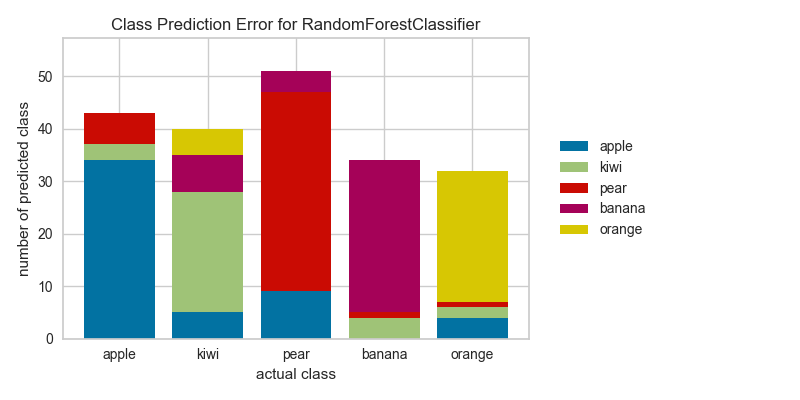# Class Prediction Error¶

The class prediction error chart provides a way to quickly understand how good your classifier is at predicting the right classes.

from sklearn.datasets import make_classification
from sklearn.model_selection import train_test_split

# Create classification dataset
X, y = make_classification(
n_samples=1000, n_classes=5, n_informative=3, n_clusters_per_class=1
)

classes = ["apple", "kiwi", "pear", "banana", "orange"]

# Perform 80/20 training/test split
X_train, X_test, y_train, y_test = train_test_split(X, y, test_size=0.20,
random_state=42)

from sklearn.ensemble import RandomForestClassifier

from yellowbrick.classifier import ClassPredictionError

# Instantiate the classification model and visualizer
visualizer = ClassPredictionError(
RandomForestClassifier(), classes=classes
)

# Fit the training data to the visualizer
visualizer.fit(X_train, y_train)

# Evaluate the model on the test data
visualizer.score(X_test, y_test)

# Draw visualization
g = visualizer.poof()## API Reference¶

Shows the balance of classes and their associated predictions.

class yellowbrick.classifier.class_prediction_error.ClassPredictionError(model, ax=None, classes=None, **kwargs)[source]

Bases: yellowbrick.classifier.base.ClassificationScoreVisualizer

Class Prediction Error chart that shows the support for each class in the fitted classification model displayed as a stacked bar. Each bar is segmented to show the distribution of predicted classes for each class. It is initialized with a fitted model and generates a class prediction error chart on draw.

Parameters: ax: axes the axis to plot the figure on. model: estimator Scikit-Learn estimator object. Should be an instance of a classifier, else __init__() will raise an exception. classes: list A list of class names for the legend. If classes is None and a y value is passed to fit then the classes are selected from the target vector. kwargs: dict Keyword arguments passed to the super class. Here, used to colorize the bars in the histogram.

Notes

These parameters can be influenced later on in the visualization process, but can and should be set as early as possible.

Attributes: score_ : float Global accuracy score predictions_ : ndarray An ndarray of predictions whose rows are the true classes and whose columns are the predicted classes
draw()[source]

Renders the class prediction error across the axis.

finalize(**kwargs)[source]

Finalize executes any subclass-specific axes finalization steps. The user calls poof and poof calls finalize.

score(X, y, **kwargs)[source]

Generates a 2D array where each row is the count of the predicted classes and each column is the true class

Parameters: X : ndarray or DataFrame of shape n x m A matrix of n instances with m features y : ndarray or Series of length n An array or series of target or class values score_ : float Global accuracy score